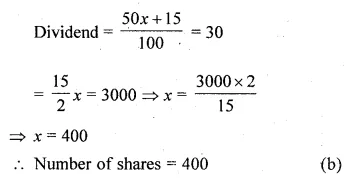## ML Aggarwal Class 10 Solutions for ICSE Maths Chapter 3 Shares and Dividends MCQS

These Solutions are part of ML Aggarwal Class 10 Solutions for ICSE Maths. Here we have given ML Aggarwal Class 10 Solutions for ICSE Maths Chapter 3 Shares and Dividends MCQS

More Exercises

Question 1.
If Jagbeer invest ₹10320 on ₹100 shares at a discount of ₹ 14, then the number of shares he buys is
(a) 110
(b) 120
(c) 130
(d) 150
Solution:
Investment = ₹ 10320
Face value of each share = ₹100
M.V. of each share = ₹100 – 14 = ₹86
No. of shares = $$\\ \frac { 10320 }{ 86 }$$ = 120 (b)

Question 2.
If Nisha invests ₹19200 on ₹50 shares at a premium of 20%, then the number of shares she buys is
(a) 640
(b) 384
(c) 320
(d) 160
Solution:
Investment = ₹19200
Face value of each share = ₹50
M.V. = ₹50 x $$\\ \frac { 120 }{ 100 }$$ = ₹60
Number of shares = $$\\ \frac { 19200 }{ 60 }$$
= 320 (c)

Question 3.
₹40 shares of a company are selling at 25% premium. If Mr. Jacob wants to buy 280 shares of the company, then the investment required by him is
(a) ₹11200
(b) ₹14000
(c) ₹16800
(d) ₹8400
Solution:
Face value of each share = ₹40
M.V. = 40 x $$\\ \frac { 125 }{ 100 }$$= ₹50
Number of shares = 280
Total investment = ₹280 x 50 = ₹ 14000 (d)

Question 4.
Arun possesses 600 shares of ₹25 of a company. If the company announces a dividend of 8%, then Arun’s annual income is
(a) ₹48
(b) ₹480
(c) ₹600
(d) ₹1200
Solution:
Number of shares = 600
F.V. of each share = ₹25
Rate of dividend = 8%
Annual income = 600 x 25 x $$\\ \frac { 8 }{ 100 }$$
= ₹1200 (d)

Question 5.
A man invests ₹24000 on ₹60 shares at a discount of 20%. if the dividend declared by the company is 10%, then his annual income is
(a) ₹3000
(b) ₹2880
(c) ₹ 1500
(d) 1440
Solution:
Investment = ₹24000
F.V. of each share = ₹60
M.V. at discount of 20% = 60 x $$\\ \frac { 80 }{ 100 }$$= ₹48
Rate of dividend = 10%Question 6.
Salman has some shares of ₹50 of a company paying 15% dividend. If his annual income is ₹3000, then the number of shares he possesses is
(a) 80
(b) 400
(c) 600
(d) 800
Solution:
F.V. of each share = ₹50
Dividend = 15%
Annual income = ₹3000
Let x be the share, then
F.V. of shares = x × 50 = ₹50xQuestion 7.
₹25 shares of a company are selling at ₹20. If the company is paying a dividend of 12%, then the rate of return is
(a) 10%
(b) 12%
(c) 15%
(d) 18%
Solution:
F.V. of each share = ₹25 ,
M.V. = ₹20
Rate of dividend = 12%
Dividend on each share = $$\\ \frac { 12 }{ 100 }$$ x 25 = ₹3
Return on ₹20 = ₹3
and on ₹100 = ₹ $$\\ \frac { 3 }{ 20 }$$ x $$\\ \frac { 5 }{ 100 }$$ = 15% (c)

Hope given ML Aggarwal Class 10 Solutions for ICSE Maths Chapter 3 Shares and Dividends MCQS are helpful to complete your math homework.

If you have any doubts, please comment below. Learn Insta try to provide online math tutoring for you.